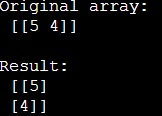# How does NumPy's transpose() method permute the axes of an array?

Learn, how does NumPy's transpose() method permute the axes of an array?
Submitted by Pranit Sharma, on January 07, 2023

NumPy is an abbreviated form of Numerical Python. It is used for different types of scientific operations in python. Numpy is a vast library in python which is used for almost every kind of scientific or mathematical operation. It is itself an array which is a collection of various methods and functions for processing the arrays.

## Permuting the axes of an array using NumPy's transpose() method

In NumPy, we have a method called transpose(), When we pass a tuple of integers to the transpose() function, the work behind it is not very complex to understand. We need to understand how NumPy transforms the array when we pass the tuple of axes.

To transpose an array, NumPy just swaps the shape and stride information for each axis.

The transpose operation swapped the strides for axis 0 and axis 1. The lengths of these axes were also swapped (both lengths are 2 in this example).

No data needs to be copied for this to happen; NumPy can simply change how it looks at the underlying memory to construct the new array.

Basically, it returns an array with axes transposed. For a 1-D array, this returns an unchanged view of the original array but for a 2-D array, this is the standard matrix transpose.

Let us understand with the help of an example,

## Python code for NumPy's transpose() method permute the axes of an array

```# Import numpy
import numpy as np

# Creating numpy array
arr = np.array([5,4])[np.newaxis]

# Display original array
print("Original array:\n",arr,"\n")

# Transposing the array
tr = arr.T

# Display result
print("Result:\n",tr)
```

Output: